# RD Sharma Solutions For Class 12 Maths Exercise 19.9 Chapter 19 Indefinite Integrals

RD Sharma Solutions for Class 12 Exercise 19.9 Chapter 19 Indefinite Integrals is provided here. This exercise gives an overview of determining the integrals of the form first-order derivative and n-th order derivative by using the substitution method using standard trigonometric identities. For students who face difficulty in understanding the concepts during class hours, the experts at BYJU’S have developed the solutions based on the students’ grasping abilities.

Students can solve both chapter-wise and exercise wise problems to increase their confidence level before appearing for the board exam. To boost interest among students in this chapter, RD Sharma Solutions for Class 12 Chapter 19 Indefinite Integrals Exercise 19.9, pdf links are given here for easy access.

## Download the PDF of RD Sharma Solutions For Class 12 Chapter 19 – Indefinite Integrals Exercise 19.9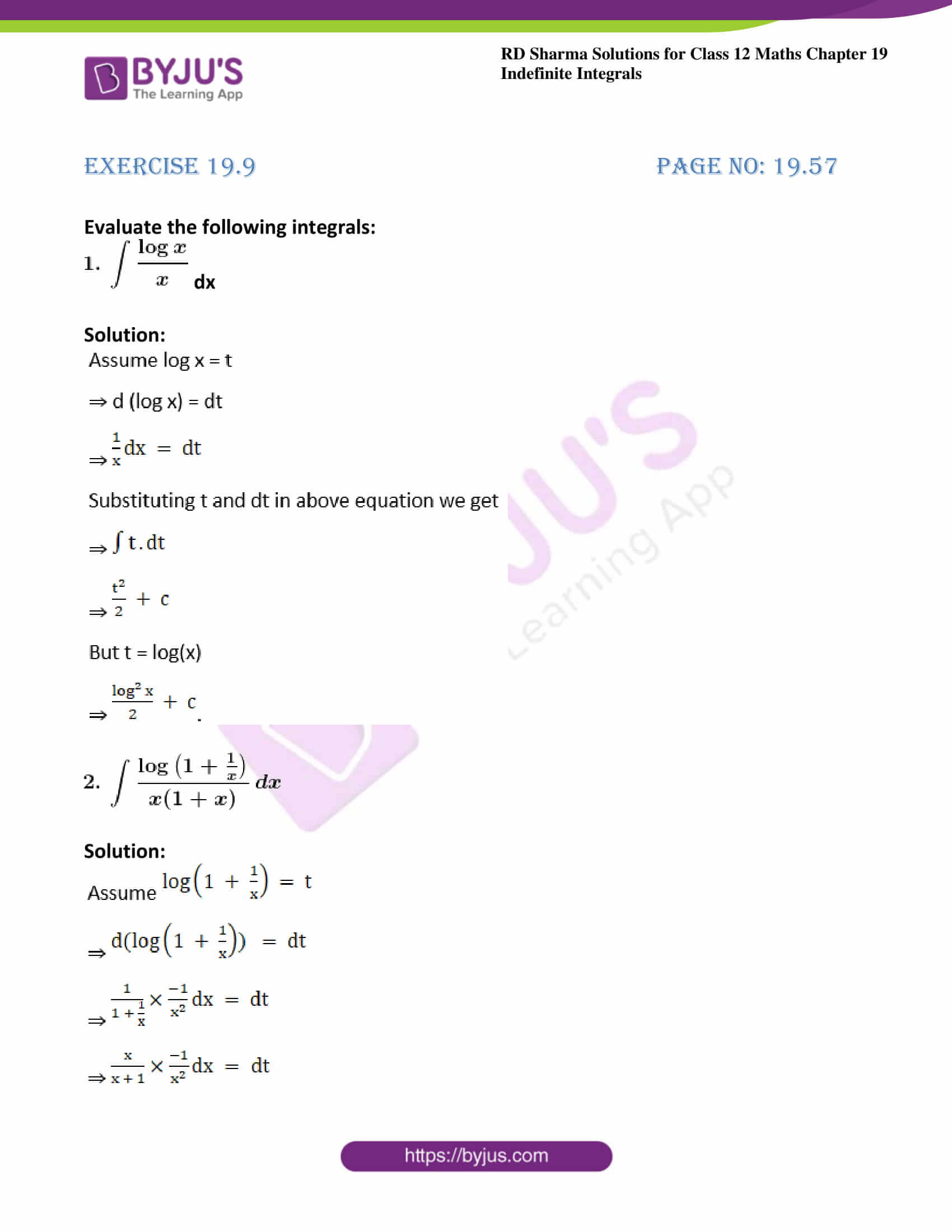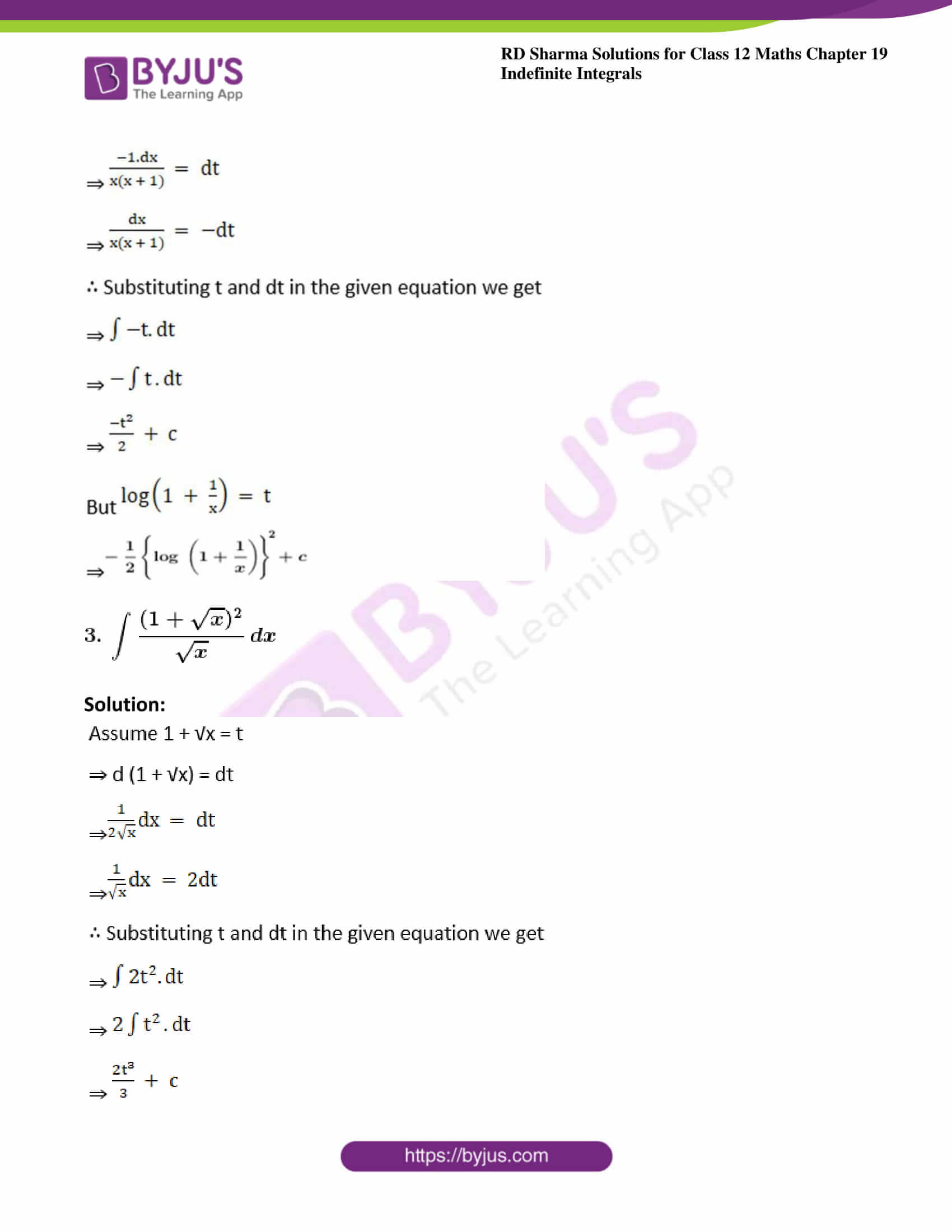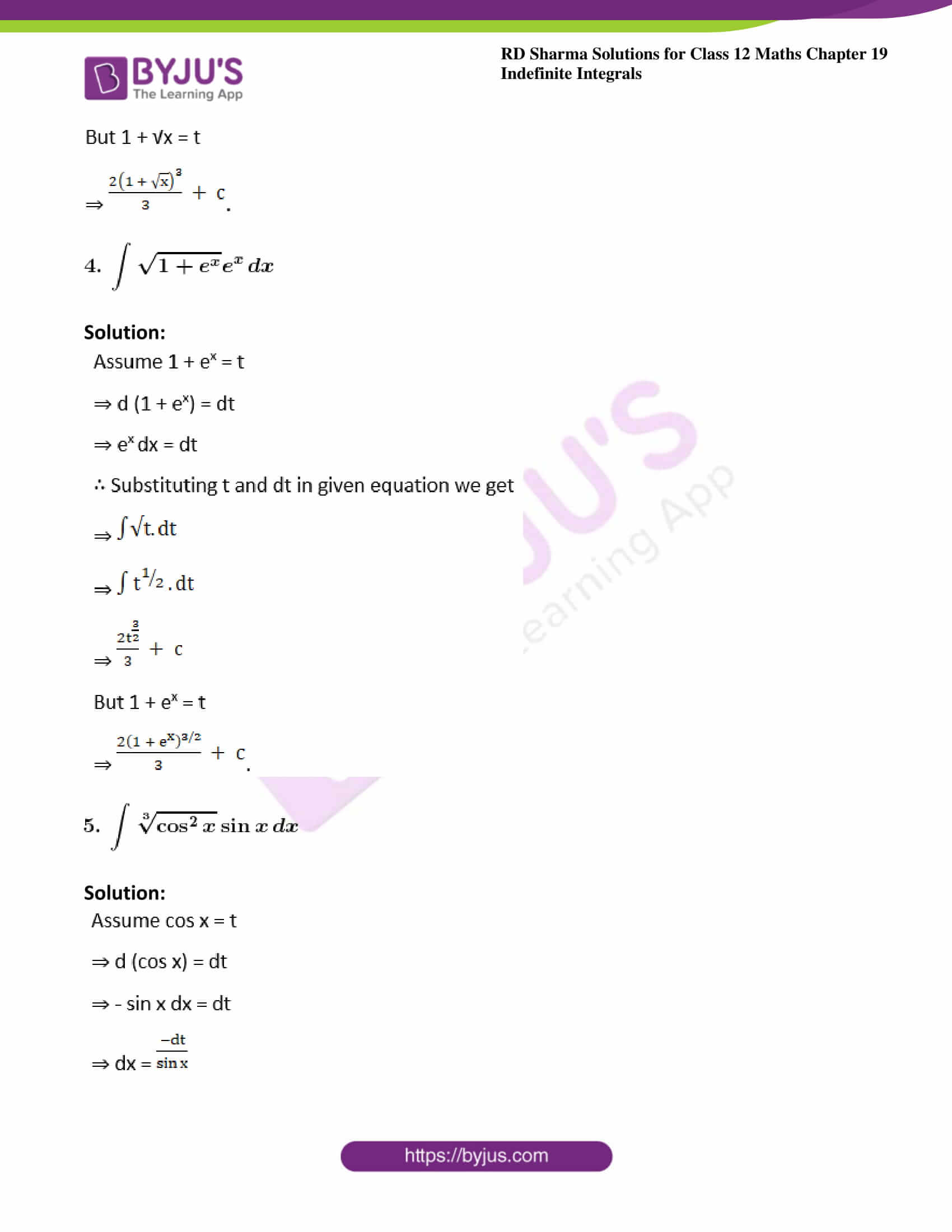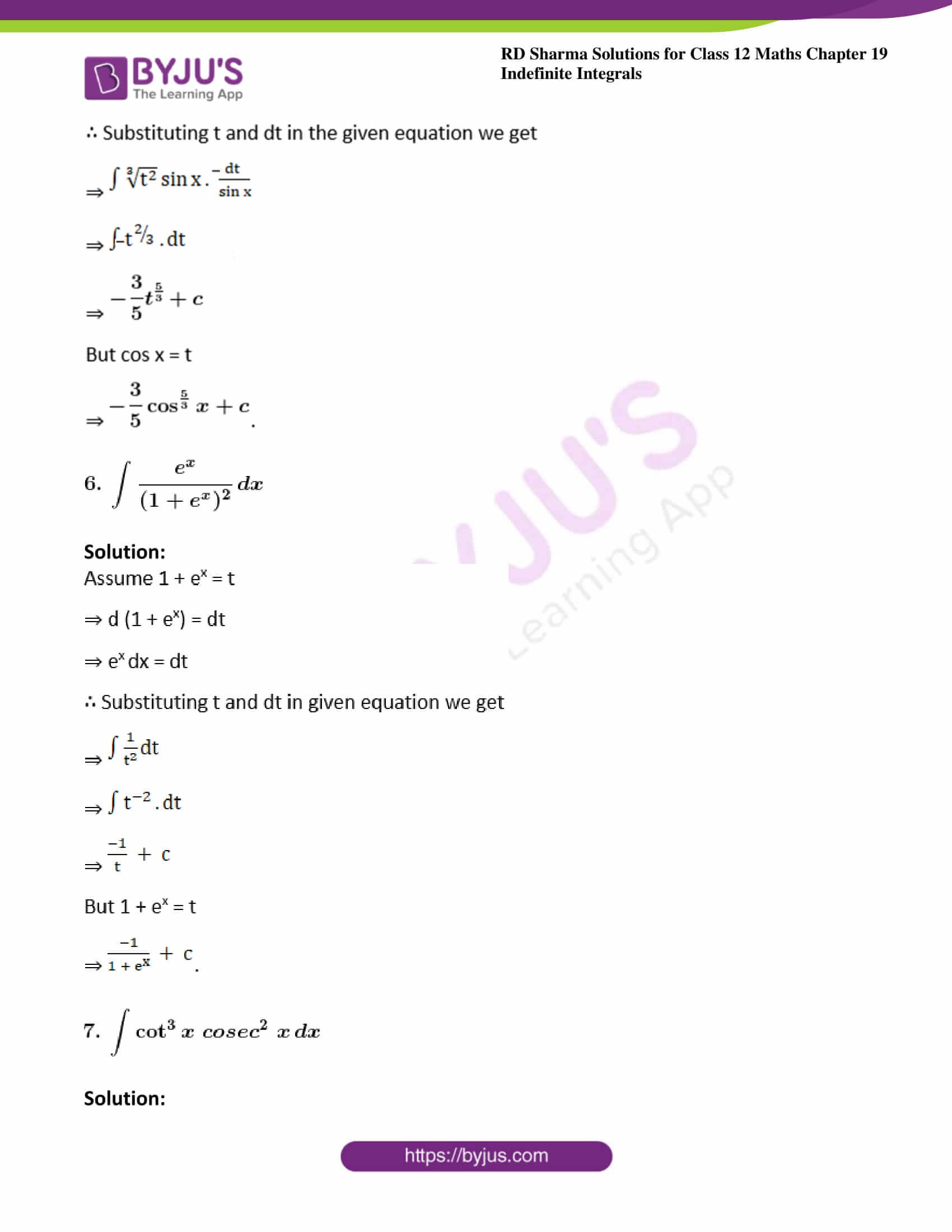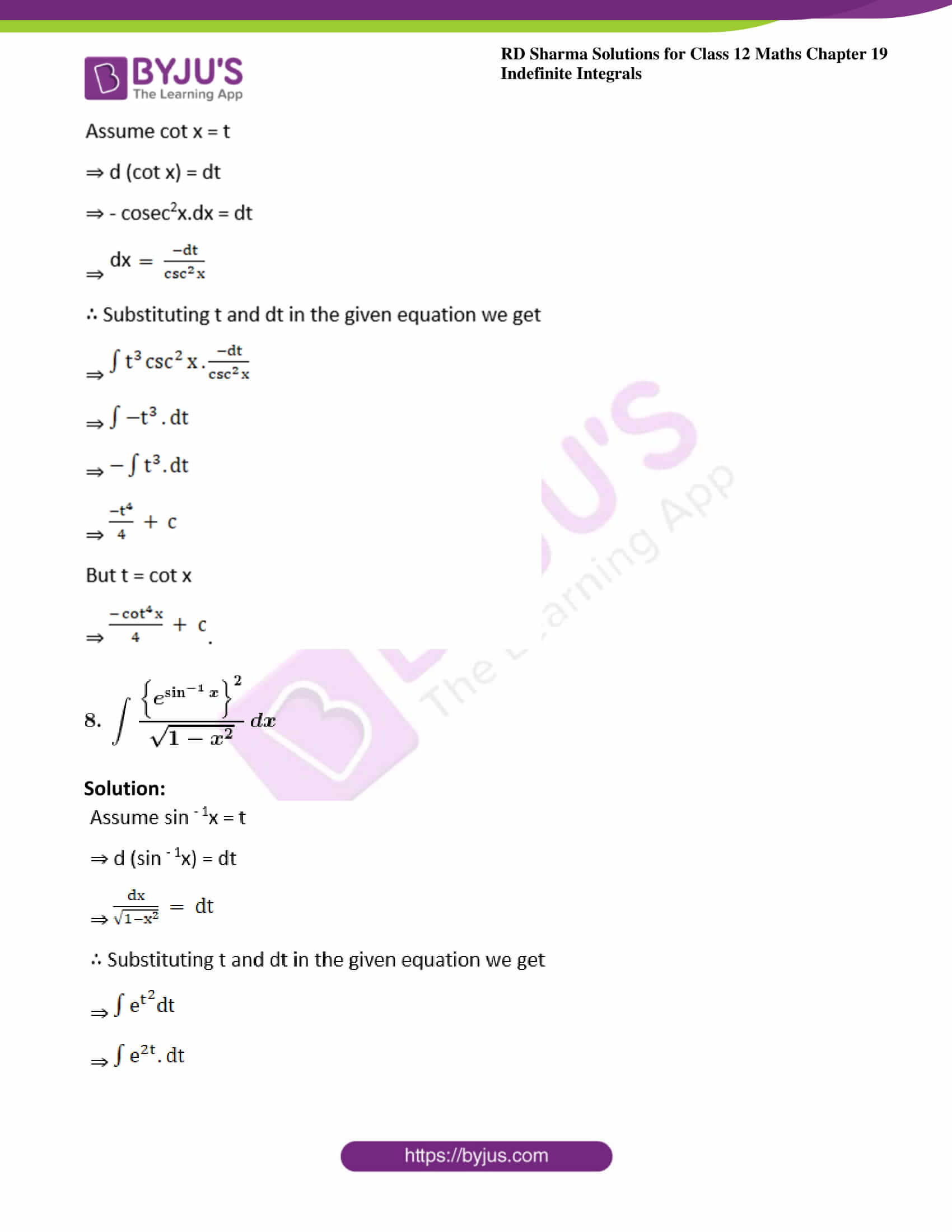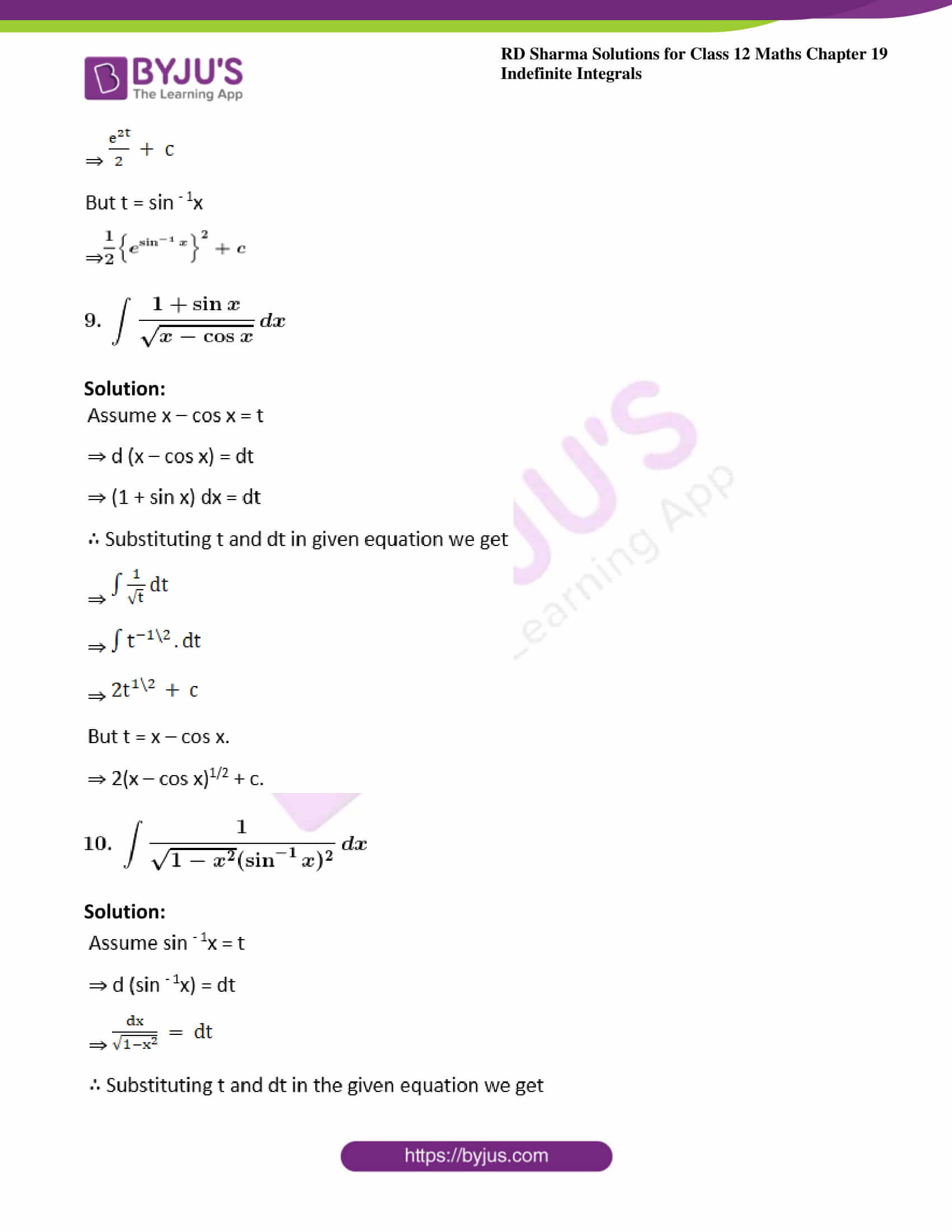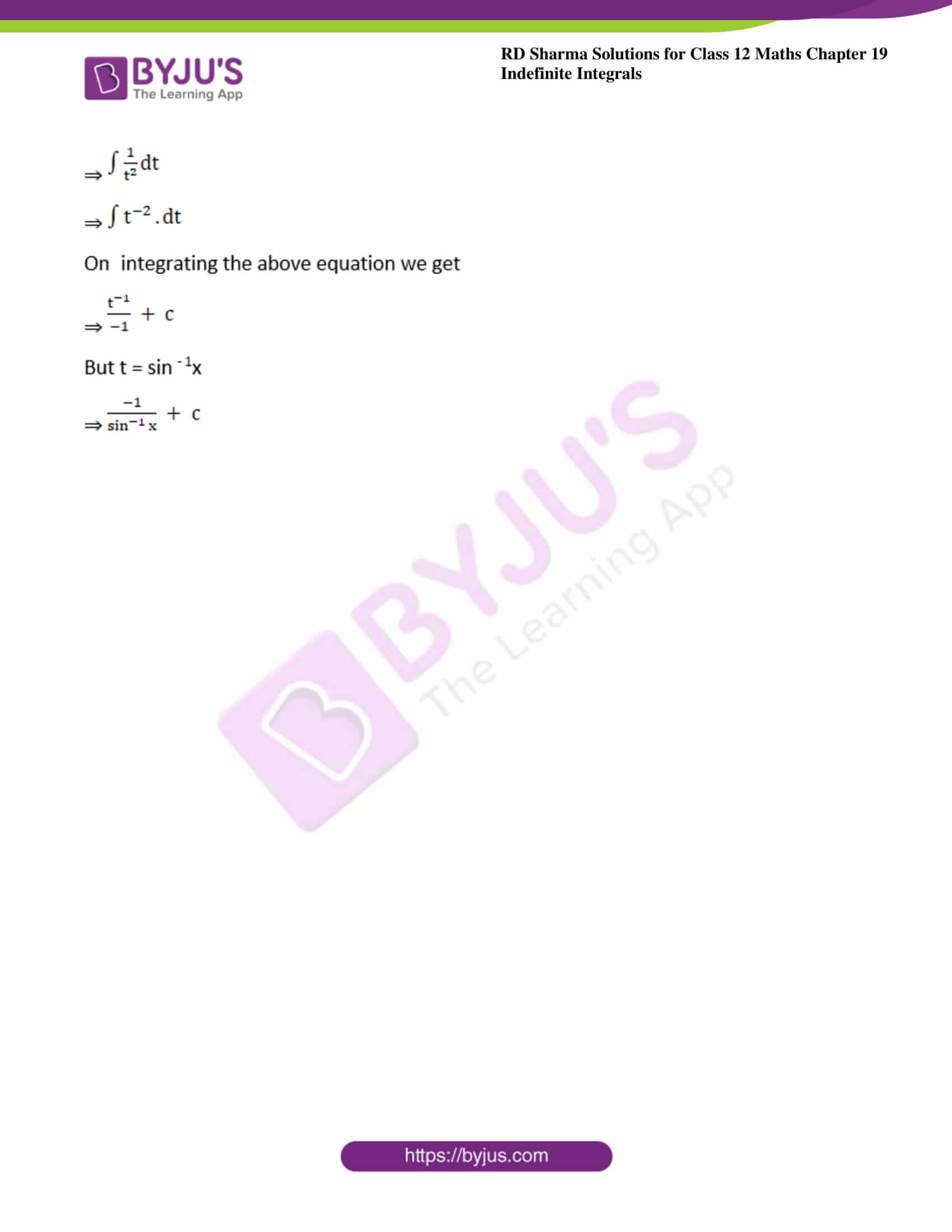### Exercise 19.9 Page No: 19.57

Evaluate the following integrals: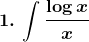dx

Solution: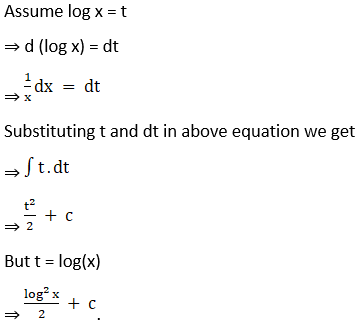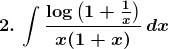Solution: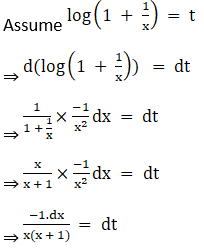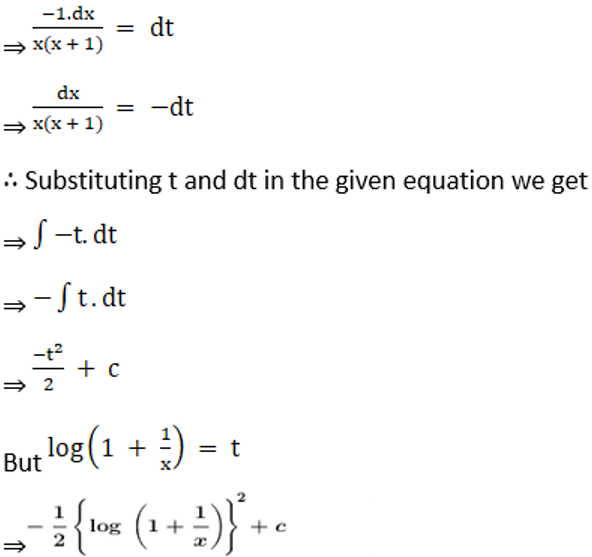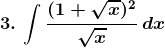Solution: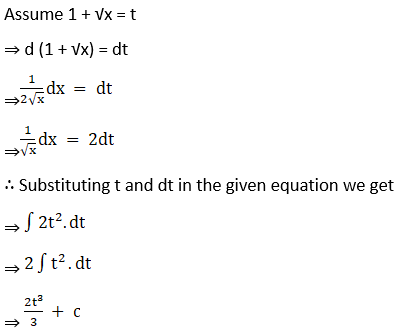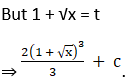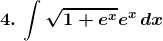Solution: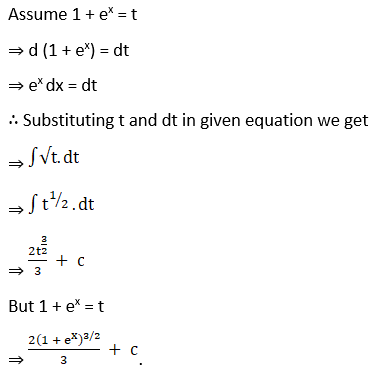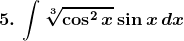Solution: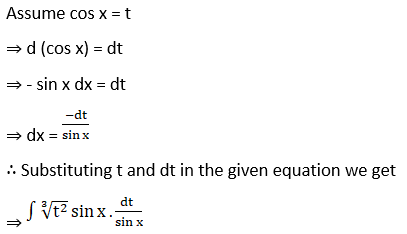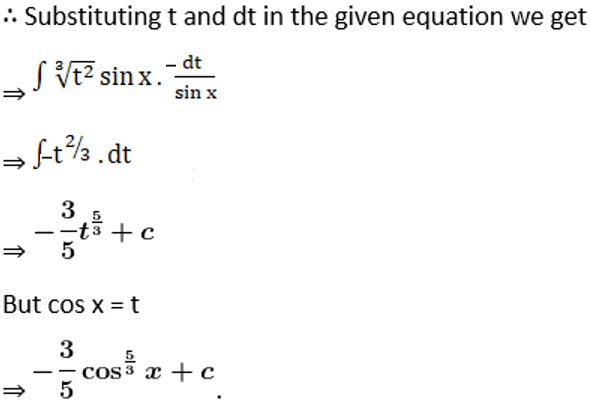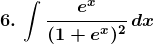Solution: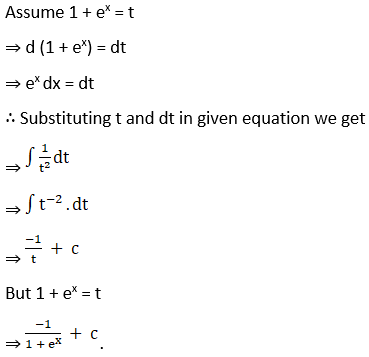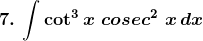Solution: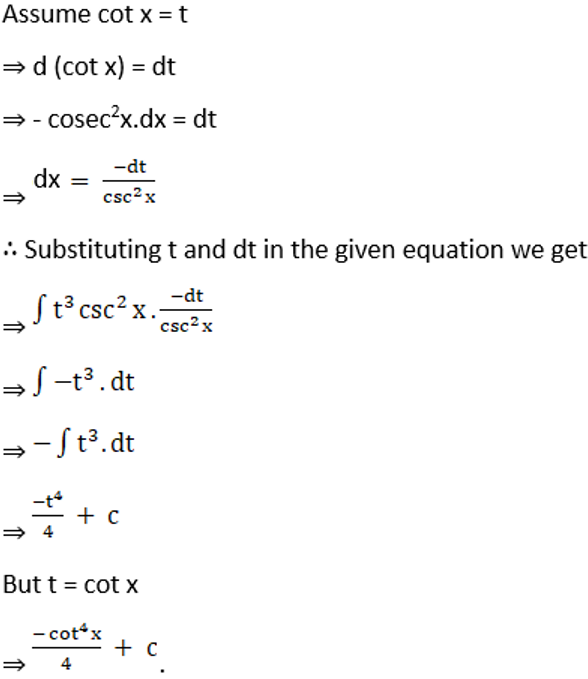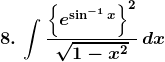Solution: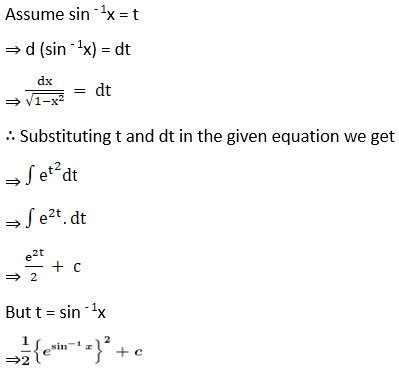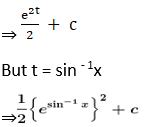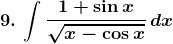Solution: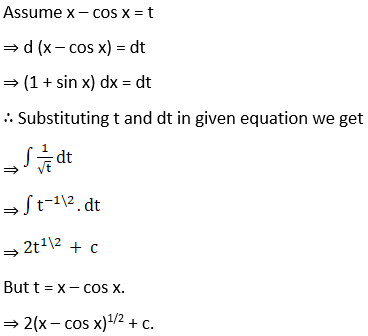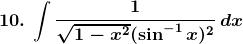Solution: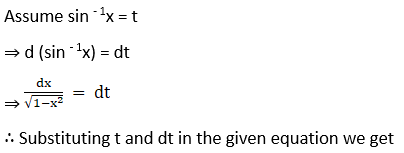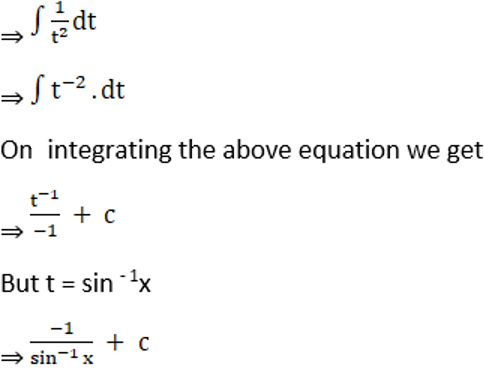### Access other exercises of RD Sharma Solutions For Class 12 Chapter 19 – Indefinite Integrals

Exercise 19.1 Solutions

Exercise 19.2 Solutions

Exercise 19.3 Solutions

Exercise 19.4 Solutions

Exercise 19.5 Solutions

Exercise 19.6 Solutions

Exercise 19.7 Solutions

Exercise 19.8 Solutions

Exercise 19.10 Solutions

Exercise 19.11 Solutions

Exercise 19.12 Solutions

Exercise 19.13 Solutions

Exercise 19.14 Solutions

Exercise 19.15 Solutions

Exercise 19.16 Solutions

Exercise 19.17 Solutions

Exercise 19.18 Solutions

Exercise 19.19 Solutions

Exercise 19.20 Solutions

Exercise 19.21 Solutions

Exercise 19.22 Solutions

Exercise 19.23 Solutions

Exercise 19.24 Solutions

Exercise 19.25 Solutions

Exercise 19.26 Solutions

Exercise 19.27 Solutions

Exercise 19.28 Solutions

Exercise 19.29 Solutions

Exercise 19.30 Solutions

Exercise 19.31 Solutions

Exercise 19.32 Solutions